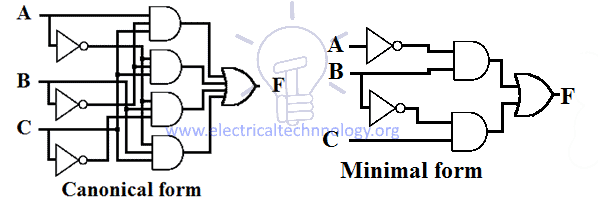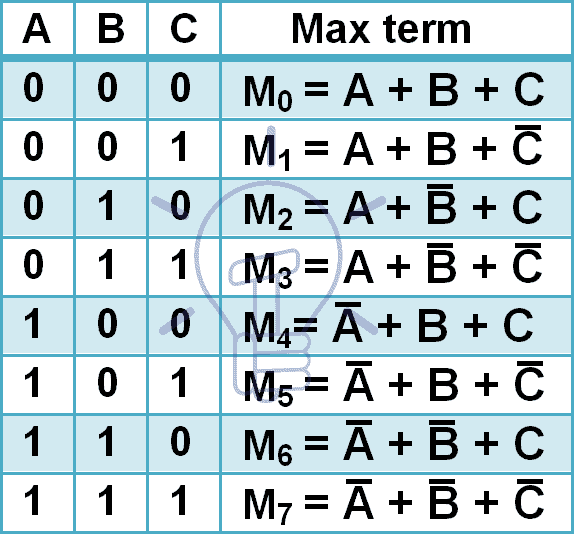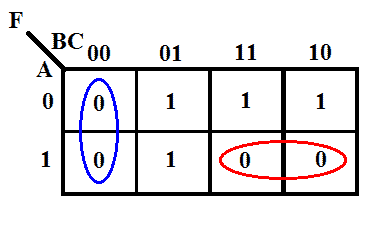# Sum Of Product (SOP) & Product Of Sum (POS)

## Product Of Sum (POS) & Sum Of Product (SOP)

### Sum Of Product (SOP)

Sum of Product is the abbreviated form of SOP. Sum of product form is a form of expression in Boolean algebra in which different product terms of inputs are being summed together. This product is not arithmetical multiply but it is Boolean logical AND and the Sum is Boolean logical OR.

To understand better about SOP, we need to know about min term.

#### Min Term

Minterm means the term that is true for a minimum number of combination of inputs. That is true for only one combination of inputs.

Since AND gate also gives True only when all of its inputs are true so we can say min terms are AND of input combinations like in the table given below.3 inputs have 8 different combinations. Each combination has a min terms denoted by small m and its decimal combination number written in subscript. Each of these minterms will be only true for the specific input combination.

#### Types of Sum Of Product (SOP) Forms

There are few different forms of Sum of Product.

• Canonical SOP Form
• Non-Canonical SOP Form
• Minimal SOP Form
##### Canonical SOP Form

This is the standard form of Sum of Product. It is formed by O Ring the minterms of the function for which the output is true. This is also known as Sum of Min terms or Canonical disjunctive normal form (CDNF). It is just a fancy name. “canonical” means “standardized” and “disjunctive” means “Logical OR union”.

Canonical SOP expression is represented by summation sign and minterms in the braces for which the output is true.

For example, a functions truth table is given below.For this function the canonical SOP expression is

F = ∑( m1, m2, m3, m5 )

Which means that the function is true for the min terms {1, 2, 3, 5}.

By expanding the summation we get.

F = m1 + m2 + m3 + m5

Now putting min terms in the expression

F = A̅B̅C + A̅BC̅ + A̅BC + AB̅C

Canonical form contains all inputs either complemented or non-complemented in its product terms.

You may also read: Digital Synchronous Counter – Types, Working & Applications

##### Non-Canonical SOP Form

As the name suggests, this form is the non-standardized form of SOP expressions. The product terms are not the min terms but they are simplified. Let’s take the above function in canonical form as an example.

F = A̅B̅C + A̅BC̅ + A̅BC + AB̅C

F = A̅B̅C + A̅B(C̅ + C) + AB̅C

F = A̅B̅C + A̅B(1) + AB̅C

F = A̅B̅C + A̅B + AB̅C

This expression is still in Sum of Product form but it is non-canonical or non-standardized form.

##### Minimal SOP Form

This form is the most simplified SOP expression of a function. It is also a form of non-canonical form. Minimal SOP form can be made using Boolean algebraic theorems but it is very easily made using Karnaugh map (K-map).

Minimal SOP form is preferred because it uses the minimum number of gates and input lines. it is commercially beneficial because of its compact size, fast speed, and low fabrication cost.

Let’s take an example of the function given above in canonical form.Its K-map is given below.According to the K-map, the output expression will be

F = B̅C + A̅B

This is the most simplified & optimized expression for the said function. This expression requires only two 2-input AND gates & one 2-input OR gate. However, the canonical form needs four 3-input AND gates & one 4-input OR gate, which is relatively more costly than minimal form implementation.

#### Schematic Design of Sum Of Product (SOP)

SOP expression implements 2 level AND-OR design in which the 1st level gate is AND gate following the 2nd level gate which is OR gate. Schematic design of SOP expression needs a group array of AND gates & one OR gate.

Every SOP expression has somewhat same designing i.e. all the inputs goes through AND gate and then the output of these AND gates flow through an OR gate as shown in the figure given below.

The number of inputs and the number of AND gates depend upon the expression one is implementing.

Example of designs of canonical and minimal SOP expression for a function is given below.#### Conversion from Minimal SOP to Canonical SOP Form

Conversion from minimal or any sort of non-canonical form to canonical form is very simple.

As we know canonical form has min terms & min terms consists of all inputs either complemented or non-complemented. So we will multiply every term of minimal SOP with the sum of missing input’s complemented and non-complemented form. Example of conversion for the above function in minimal SOP form is given below.

Minimal SOP form

F = A̅B + B̅C

The term A̅B is missing input C. So we will multiply A̅B with (C+C̅) because (C+C̅ = 1). The term B̅C is missing input A. so it will be multiplied with (A+A̅)

F = A̅B(C + C̅) + B̅C(A + A̅)

F = A̅BC + A̅BC̅ + AB̅C + A̅B̅C

Now, this expression is in canonical form.

#### Conversion from Canonical SOP to Canonical POS

Standard SOP expression can be converted into standard POS (product of sum) expression. For example, the function given above is in canonical SOP form

F = A̅B̅C + A̅BC̅ + A̅BC + AB̅C

F = ∑ ( m1, m2, m3, m5 )

The remaining terms of this function are maxterms for which output is false. These max terms are M0,M4,M6,M7. These Max terms will be used in POS expression as the product of these max terms. The Symbol of Product is ∏.

F = ∏ ( M0, M4, M6, M7)

F = (A+B+C)(A̅+B+C)(A̅+B̅+C)(A̅+B̅+C̅)

The Max terms are the complement of minterms. Which is why M0=(A+B+C).

#### Conversion from Canonical SOP to Minimal SOP

Canonical SOP can be converted to minimal SOP. It can be converted using Karnaugh map or Boolean algebraic theorems. The K-map method is very easy and its example has been done above in the minimal SOP form.

### Product of Sum

Product of Sum abbreviated for POS.

The product of Sum form is a form in which products of different sum terms of inputs are taken. These are not arithmetic product and sum but they are logical Boolean AND and OR respectively.

To better understand about Product of Sum, we need to know about Max term.

#### Max Term

Maxterm means the term or expression that is true for a maximum number of input combinations or that is false for only one combination of inputs.

Since OR gate also gives false for only one input combination. So Maxterm is OR of either complemented or non-complemented inputs.

Max terms for 3 input variables are given below.3 inputs have 8 different combinations so it will have 8 maxterms. Maxterms are denoted by capital M and decimal combination number In the subscript as shown in the table given above.

In maxterm, each input is complemented because Maxterm gives ‘0’ only when the mentioned combination is applied and Maxterm is complement of minterm.

M3               =             m̅3

M3               =             (A̅BC)’

M3               =             A + B̅ +C̅               DE Morgan’s law

Which is why for A=0 Max term consist A & for A=1 Max term consist A̅.

You may also read: Digital Flip-Flops – SR, D, JK and T Flip Flops

#### Types of Product Of Sum Forms

There are different types of Product of Sum forms.

• Canonical POS Form
• Non – Canonical Form
• Minimal POS Form
##### Canonical POS Form

It is also known as Product of Max term or Canonical conjunctive normal form (CCNF). Canonical means standard and conjunctive means intersection.

In this form, Maxterms are AND together for which output is false.

Canonical POS expression is represented by ∏ and Maxterms for which output is false in brackets as shown in the example given below.F              =             ∏ (M0, M4, M6, M7)

Expanding the product

F              =             M0.M4.M6.M7

Putting Max terms

F              =             (A+B+C)(A̅+B+C)(A̅+B̅+C)(A̅+B̅+C̅)

The canonical form contains all inputs either complemented or non-complemented in its each Sum term.

##### Non – Canonical Form

The product of sum expression that is not in standard form is called non-canonical form.

Let’s take the above-given function as an example.

F              =             (A+B+C)(A̅+B+C)(A̅+B̅+C)(A̅+B̅+C̅)

F              =             (B+C) (A̅+B̅+C)(A̅+B̅+C̅)

Same but inverted terms eliminates from two Max terms and form a single term to prove it here is an example.

= (A+B+C) (A̅+B+C)

= AA̅+AB+AC+A̅B+BB+BC+A̅C+BC+CC

= 0+AB+AC+A̅B+A̅C+B+BC+C

= A(B+C)+A̅(B+C)+B(1+C)+C

= (B+C)(A+A̅)+B(1)+C

= (B+C)(0)+B+C

= B+C

The expression achieved is still in Product of Sum form but it is non-canonical form.

##### Minimal POS Form

This is the most simplified and optimized form of a POS expression which is non-canonical. Minimal Product of Sum form can be achieved using Boolean algebraic theorems like in the non-canonical example given above. Another method of achieving minimal POS form is by using Karnaugh map which is comparatively easier than using Boolean algebraic theorems.

Minimal POS form uses less number of inputs and logic gates during its implementation, that’s why they are being preferred over canonical form for their compact,fast and low-cost implementation.

Let’s take the above-given function as example

K-map of the functionMinimal expression using K-map

F = (B+C) (A̅+B̅)

The achieved expression is the minimal product of sum form. It is still Product of Sum expression But it needs only 2 inputs two OR gates and a single 2 input AND gate. However, the canonical form needs 4 OR gates of 3 inputs and 1 AND gate of 4 inputs.

• You may also read: Negative Feedback and Negative Feedback Amplifier Systems

#### Schematic Design of Product of Sum (POS)

The product of Sum expression has a specific schematic design of OR-AND. In OR-AND the inputs go through an array of OR gates which is the first level of gates, the output of the first level OR gates goes through the second level of the gate,which is an AND gate.

The number of inputs and number of gates used in this design depends upon the expression that is to be implemented.

The canonical form consists of the max number of possible inputs and gates,however, the minimal form consists of the lowest possible number of inputs and gates. The schematic design of canonical and minimal POS form is given below.#### Conversion from Minimal POS to Canonical form POS

As we know the canonical form of POS has max terms and max terms contains every input either complemented or non-complemented. So we will add every sum term with the product of complemented and non-complemented missing input. Example of its conversion is given below.

Minimal POS form

F = (A̅+B̅) (B+C)

(A̅+B̅) term is missing C input so we will add (CC̅) with it. (B+C) term is missing A input so we will add (AA̅) with it.

F = (A̅+B̅+CC̅) (B+C+AA̅)

F = (A̅+B̅+C)(A̅+B̅+C̅)(A+B+C)(A̅+B+C)

This expression is now in canonical form.

• You may also read: Counter and Types of Electronic Counters

#### Conversion From Canonical POS to SOP

The product of Sum expression can be converted into Sum of Product form only if the expression is in canonical form. Canonical POS and canonical SOP are inter-convertible i.e. they can be converted into one another.  Example of POS to SOP conversion is given below.

POS canonical form

F = (A+B+C)(A̅+B+C)(A̅+B̅+C)(A̅+B̅+C̅)

In canonical form each sum term is a max term so it can also be written as:

F = ∏(M0,M4,M6,M7)

The remaining combinations of inputs are minterms of the function for which its output is true. To convert it into SOP expression first we will change the symbol to summation (∑) and use the remaining minterm.

F = ∑ (m1,m2,m3,m5)

Now we will expand the summation sign to form canonical SOP expression.

F = A̅B̅C + A̅BC̅ + A̅BC + AB̅C

Min terms are complement of Max terms for the same combination of inputs.

#### Canonical to Minimal POS

A canonical Product of Sum expression can be converted into Minimal Product of sum form by using Karnaugh map (K-map). Another method for converting canonical into minimal is by using Boolean algebraic theorems.

The use of K-map is very easy that is why K-map is preferred. For minimal POS expression, 0’s in K-map are combined into groups and the expression we get is complemented since the groups were made of ‘0’s. Its example has been done above.